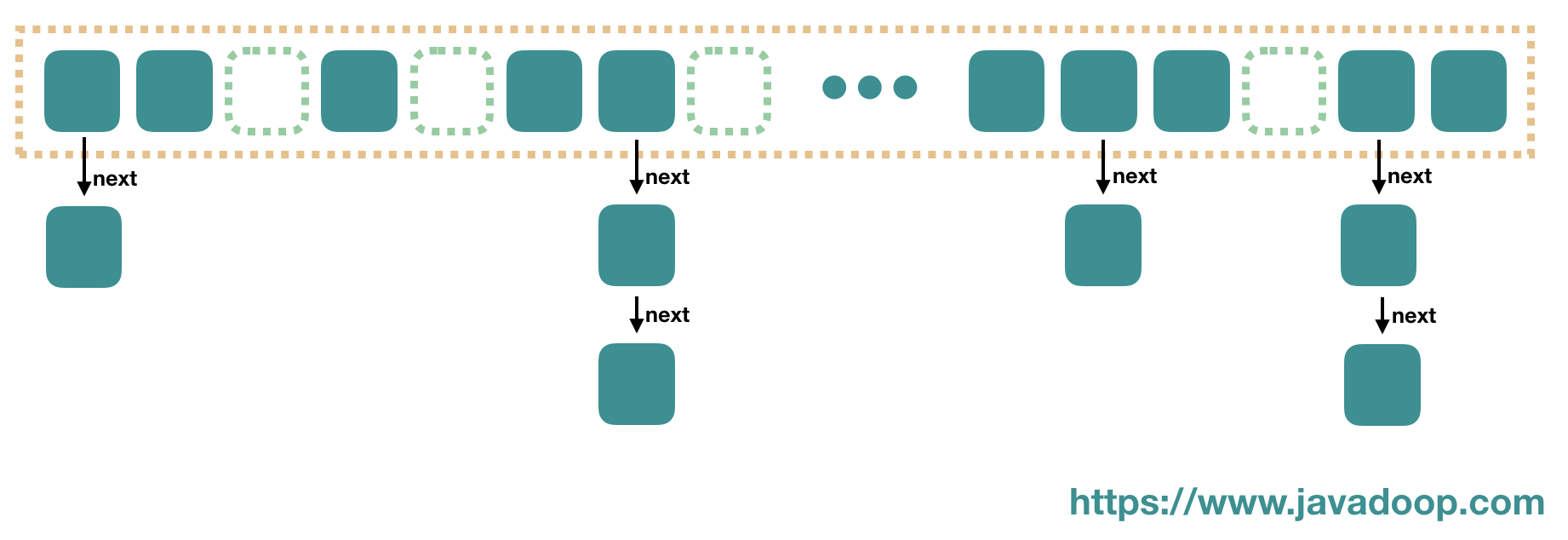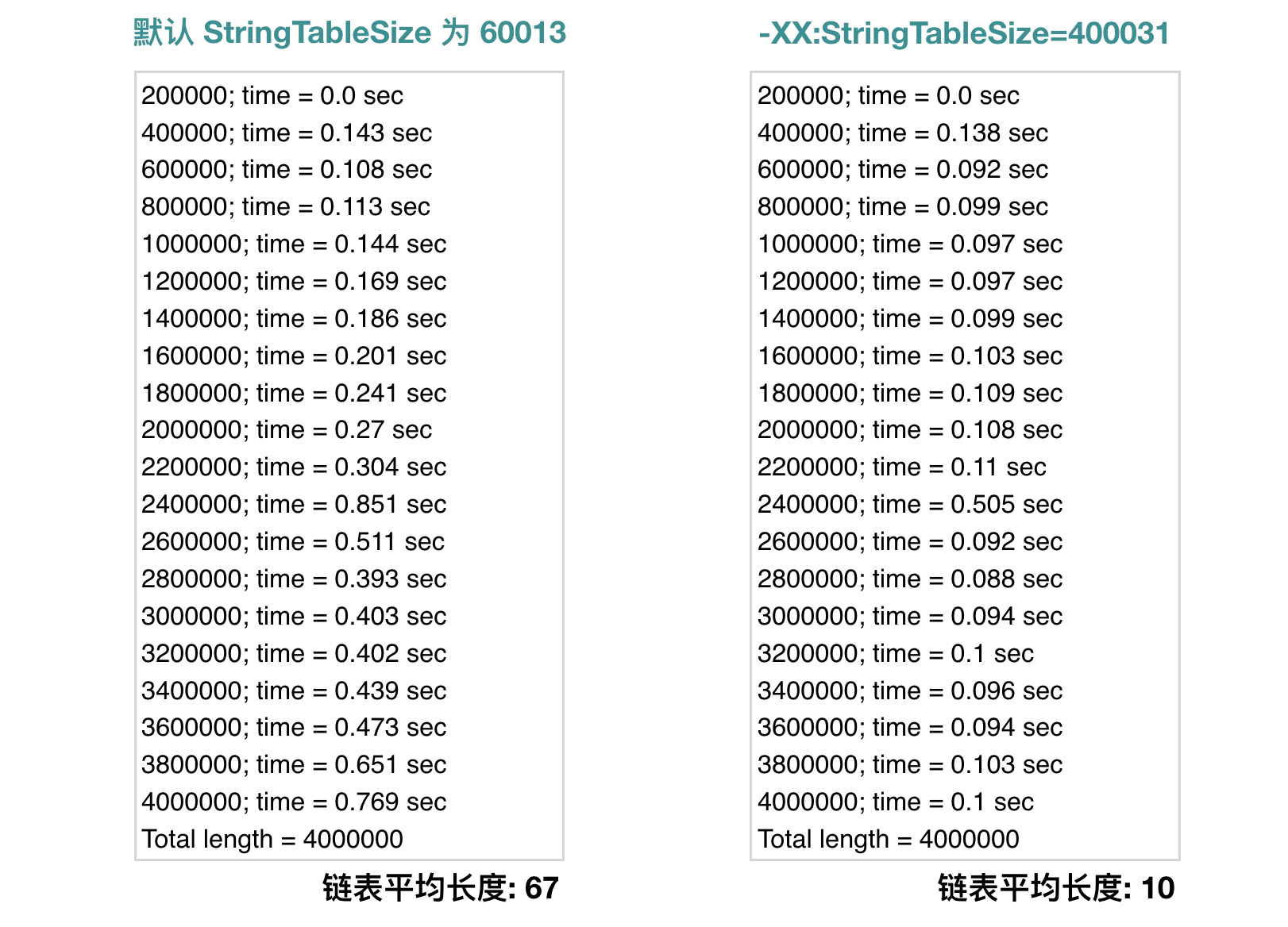Integer 可通过设置 java.lang.Integer.IntegerCache.high 扩大缓存区间

String 不是基础类型，但是它也有同样的机制，通过 String Pool 来缓存 String 对象。假设 "Java" 这个字符串我们会在应用程序中使用多次，我们肯定不希望在每次使用到的时候，都重新在堆中创建一个新的对象。## 创建和回收

• 如果字符串未在 Pool 中，那么就往 Pool 中增加一条记录，然后返回 Pool 中的引用。
• 如果已经在 Pool 中，直接返回 Pool 中的引用。

## 讨论 String Pool 的实现

1、首先，我们先考虑 String Pool 的空间问题。

2、其次，我们再讨论 String Pool 的实现问题。

JVM 内部的 HashTable 是不扩容的，但是不代表它不 rehash，它会在发现散列不均匀的时候进行 rehash，这里不展开介绍。

3、观察 String Pool 的使用情况。

JVM 提供了 `-XX:+PrintStringTableStatistics` 启动参数来帮助我们获取统计数据。

``````SymbolTable statistics:
Number of buckets       :     20011 =    160088 bytes, avg   8.000
Number of entries       :     10923 =    262152 bytes, avg  24.000
Number of literals      :     10923 =    425192 bytes, avg  38.926
Total footprint         :           =    847432 bytes
Average bucket size     :     0.546
Variance of bucket size :     0.545
Std. dev. of bucket size:     0.738
Maximum bucket size     :         6
## 看下面这部分：
StringTable statistics:
Number of buckets       :     60003 =    480024 bytes, avg   8.000
Number of entries       :   4000774 =  96018576 bytes, avg  24.000
Number of literals      :   4000774 = 1055252184 bytes, avg 263.762
Total footprint         :           = 1151750784 bytes
Average bucket size     :    66.676
Variance of bucket size :    19.843
Std. dev. of bucket size:     4.455
Maximum bucket size     :        84

``````

``````StringTable statistics:
Number of buckets       :   60003
Average bucket size     :      67
Variance of bucket size :      20
Std. dev. of bucket size:       4
Maximum bucket size     :      84
``````

## 测试 String Pool 的性能

``````package com.javadoop;

import java.lang.ref.WeakReference;
import java.util.ArrayList;
import java.util.List;
import java.util.WeakHashMap;

public class StringTest {

public static void main(String[] args) {
test(4000000);
}

private static void test(int cnt) {
final List<String> lst = new ArrayList<String>(1024);
long start = System.currentTimeMillis();
for (int i = 0; i < cnt; ++i) {
final String str = "Very very very very very very very very very very very very very very " +
"very long string: " + i;

if (i % 200000 == 0) {
System.out.println(i + 200000 + "; time = " + (System.currentTimeMillis() - start) / 1000.0 + " sec");
start = System.currentTimeMillis();
}
}
System.out.println("Total length = " + lst.size());
}
}
``````

``````# 编译
javac -d . StringTest.java
# 使用默认 table size (60013) 运行一次
# 设置 table size 为 400031，再运行一次
``````## 讨论自建 String Pool

``````private static final WeakHashMap<String, WeakReference<String>> pool
= new WeakHashMap<String, WeakReference<String>>(1024);

private static String manualIntern(final String str) {
final WeakReference<String> cached = pool.get(str);
if (cached != null) {
final String value = cached.get();
if (value != null) {
return value;
}
}
pool.put(str, new WeakReference<String>(str));
return str;
}
``````

``````private static void test(int cnt) {
final List<String> lst = new ArrayList<String>(1024);
long start = System.currentTimeMillis();
for (int i = 0; i < cnt; ++i) {
// 
// 

if (i % 200000 == 0) {
System.out.println(i + 200000 + "; time = " + (System.currentTimeMillis() - start) / 1000.0 + " sec");
start = System.currentTimeMillis();
}
}
System.out.println("Total length = " + lst.size());
}
``````

## 小结

StringTableSize，在 Java 6 中，是 1009；在 Java 7 和 Java 8 中，默认都是 60013，如果有必要请自行扩大这个值。

## 参考资料

Java Performance Tuning Guide: String.intern in Java 6, 7 and 8 – string pooling

（全文完）